Next: Capacitance Up: Capacitance Previous: Charge Storage

## Breakdown

Is there any practical limit to the chargewhich can be accumulated on the conducting sphere of a Van de Graaff generator? Well, we know that the field outside the sphere is just the same as if the chargewere placed at the centre of the sphere. In fact, the electric field is at its most intense just above the surface of the sphere, where it has the magnitude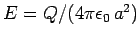. Here,is the radius of the sphere. Air (assuming that the sphere is surrounded by air) is generally a very good insulator. However, air ceases to be an insulator when the electric field-strength through it exceeds some critical value which is about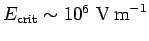. This phenomenon is known as breakdown, and is associated with the formation of sparks. The explanation of breakdown is quite straightforward. Air naturally contains a very small fraction of ionized molecules (not enough to prevent air from being an insulator). In an electric field, these ionized molecules are constantly being accelerated, and then crashing into neutral molecules. As the strength of the field is increased, the ionized molecules are accelerated to ever higher energies before crashing into the neutral molecules. Eventually, a critical field-strengthis reached at which the ionized molecules are accelerated to a sufficiently high energy that they ionize the neutral molecules when they hit them. At this point, a chain reaction takes place which rapidly leads to the almost complete ionization of the air. Thus, the air makes an almost instantaneous transition from a good insulator to a good conductor. It follows that the chargeon the conducting sphere of a Van de Graaff generator can never exceed the critical value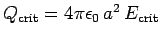, because for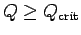the electric field around the sphere is sufficiently intense to cause breakdown. Of course, when breakdown occurs the charge on the sphere is conducted to earth.

The phenomenon of breakdown sets an upper limit on the charge which can be stored on a conductor. There is, however, another important factor which affects the onset of breakdown. This is best illustrated in the following simple example. Suppose that we have two charged conducting spheres of radiiand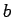, respectively, which are connected by a long conducting wire. The wire allows charge to move back and forth between the spheres until they reach the same potential (recall that the electric potential is uniform in a conductor). Let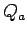be the charge on the first sphere, and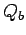the charge on the second sphere. Of course, the total charge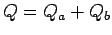carried by the two spheres is a conserved quantity. The electric field generated by each sphere is the same as if the charge on that sphere were concentrated at its centre. Assuming that the wire is sufficiently long that the two spheres do not affect one another very much, the absolute potential of the first sphere is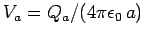, whereas that of the second sphere is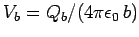[see Eq. (97)]. Since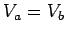, we find that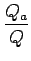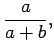(101)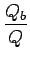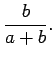(102)

Note that if the second sphere is much smaller than the first (i.e., if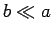) then the larger sphere grabs the lion's share of the charge: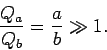(103)

The electric field-strengths just above the surfaces of the two spheres are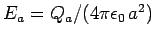and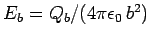, respectively. Thus, the ratio of the field-strengths generated in the immediate vicinities of the two spheres is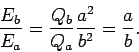(104)

Clearly, ifthen the field just above the smaller sphere is far stronger than that above the larger one. Suppose that the total charge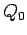on the two spheres is gradually increased until breakdown occurs. Since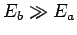, it follows that breakdown always occurs above the smaller sphere.

Equation (104) is a special case of a far more general rule: i.e., the electric field-strength above some point on the surface of a conductor is inversely proportional to the local radius of curvature of the surface. It is clear that if we wish to store significant amounts of charge on a conductor then the surface of the conductor must be made as smooth as possible. Any sharp spikes on the surface possess relatively small radii of curvature. Intense local electric fields are generated above these spikes whenever the conductor is charged. These fields can easily exceed the critical field for the breakdown of air, leading to sparking, and the eventual loss of the charge on the conductor. Sparking tends to be very destructive because of its highly localized nature, which leads inevitably to very large electric currents, and, hence, to intense heating.

Clouds can acquire very large negative charges during thunderstorms. An equal and opposite positive charge is induced on the surface of the Earth. The electric field generated between the clouds and the Earth can become sufficiently large to cause breakdown in the atmosphere, giving rise to the phenomenon which we call lightning. Let us consider the various factors which determine where lightning strikes. Breakdown starts at cloud level, as a so-called dark leader'' of ionized air traces out a path towards the ground. When it comes within about 10 meters of ground level, a second dark leader comes up from the ground to meet it. Once the two leaders meet, and a conducting path is established, the lightning strike proper occurs. Note that, contrary to popular opinion, the lightning strike travels upwards from the Earth to the clouds. It is clear that lightning strikes'' a particular object on the ground because the object emits a dark leader: i.e., because breakdown takes place just above the object. In a thunderstorm, the ground, and the objects upon it, acts essentially like a charged conductor with a convoluted surface. Thus, any spikes'' on the ground (e.g., a person standing in a field, a radio mast, a lightning rod) are comparatively more likely to be hit by lightning, because the electric field-strength above these points is relatively large, which facilitates breakdown.Next: Capacitance Up: Capacitance Previous: Charge Storage
Richard Fitzpatrick 2007-07-14